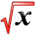# Tabular IntegrationThis article is part of the MathHelp Tutoring Wiki

Tabular integration is a method of quickly integrating by parts many times in sequence. This method requires that one of the functions in f(x)*g(x) be differentiable until it is zero. We must also be able to integrate the other function every time differentiate the first function.

To integrate with tabulation create a table of 4 columns wide. In the first column insert all of the derivatives of a function till 0. In the next column iterate the other function through integration for every non zero derivative. Finally in the third column, alternate the sign from (+) and (-). Now multiply the first cell in the table with the next two in the row below, place the result in the "term" column. This should draw a hockey stick pattern on the table. Repeat this action for every row in the table. Notice how we have to stop before we multiple the derivative of 6. To get our integrated result, simply sum all of the terms together.

Ex: Integrate x^3*Sin(x)

D I Sign Term
x^3 Sin(x) - 0
3x^2 -Cos(x) + -x^3*Cos(x)
6x -Sin(x) - 3x^2*Sin(x)
6 Cos(x) + 6x*Cos(x)
0 Sin(x) - -6*Sin(x)

Sum of terms (Ans): - x^3*Cos(x) + 3*x^2*Sin(x) + 6*x*Cos(x) - 6*Sin(x)